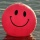Home
IT Knowledge
Inspiration
Languages
EN

# Node.js - check if path is relative

0 points
Created by:Zayaan-Rasmussen
323

In this article, we would like to show you how to check if path is relative in Node.js.

1. Import `path` module using:

``const path = require('path');``

2. Use `path.isAbsolute()` method with the path you want to check as an argument to find out if it is absolute (`true`) or relative (`false`).

Practical example:

``````const path = require('path');

// example paths
const relativePath = './index.js';
const absolutePath = 'C:/projects/app/index.js';

// check if path is absolute
const result1 = path.isAbsolute(relativePath);
const result2 = path.isAbsolute(absolutePath);

console.log(result1); // false
console.log(result2); // true``````

Output:

``````false
true``````